Browse by Topic
Related Topics

# Mathematica Q&A Series: Generating Random Numbers

Got questions about Mathematica? The Wolfram Blog has answers! We’ll regularly answer selected questions from users around the web. You can submit your question directly to the Q&A Team.

This week’s question comes from Peter, a secondary school teacher:

How can I generate random integers between -10 and 10, but excluding 0?

Read below or watch this screencast for the answer (we recommend viewing it in full-screen mode):

The built-in function RandomInteger can generate random integers with equal probability in a given range. Here are 50 random integers between -10 and 10: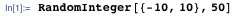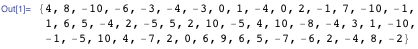But, of course, sometimes you get zero. What if you want to exclude zero?

There are several different ways to solve this problem. First, you could simply use RandomInteger as above, and then filter out all the zeroes, using a function like DeleteCases: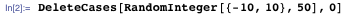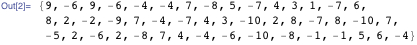The disadvantage with this approach is that you don’t know in advance how many numbers you’ll get. In this case, we got just 47 nonzero integers: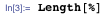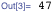For a better method, start by setting up the list of acceptable integers in advance: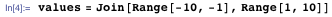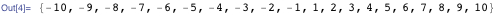Or equivalently: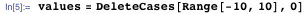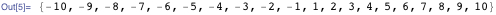Then you can use the function RandomChoice to get 50 random selections from the list: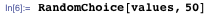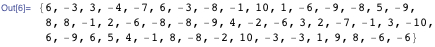This is a good way to solve this particular problem.

A different, more general approach is to begin with the fact that you want to generate random variates from a certain discrete probability distribution.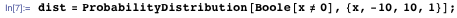(The last element, 1, in the range specification {x, -10, 10, 1} indicates a discrete distribution.)

The first argument given to ProbabilityDistribution, Boole[x ≠ 0], is a probability density function (PDF) that is zero at x = 0 and constant elsewhere: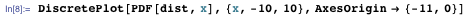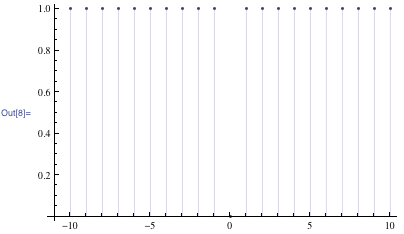Now you can use RandomVariate to generate a list of random variates from this distribution: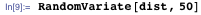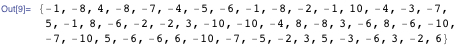This approach, using ProbabilityDistribution, is more general because you could use any logical predicate inside Boole: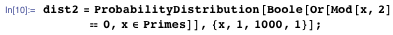This is a uniform distribution over all the integers between 1 and 1000 that are either even or prime. Generate 20 such integers: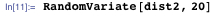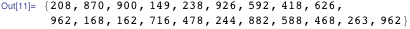There are several other built-in functions for generating random numbers that you can explore. RandomSample gives a random subset of a list, as if you were drawing numbers from a bag without replacing them: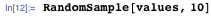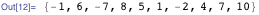Note how no number is repeated in the random sample.

RandomReal and RandomComplex can be used in a similar way as RandomInteger, to get uniformly distributed random numbers within a specific range: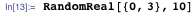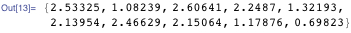And RandomVariate can be used with any probability distribution, whether it be discrete or continuous, single or multivariate. Learn more at the guide page for random number generation.

You can also download this post as a Computable Document Format (CDF) file.

If you have a question you’d like answered in this blog, you can submit it to the Q&A Team. For daily bite-sized Mathematica tips, follow our @MathematicaTip Twitter feed.